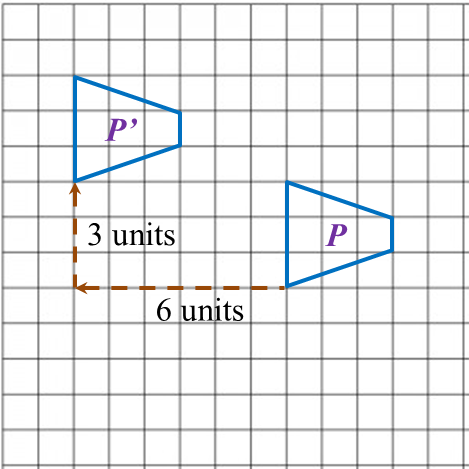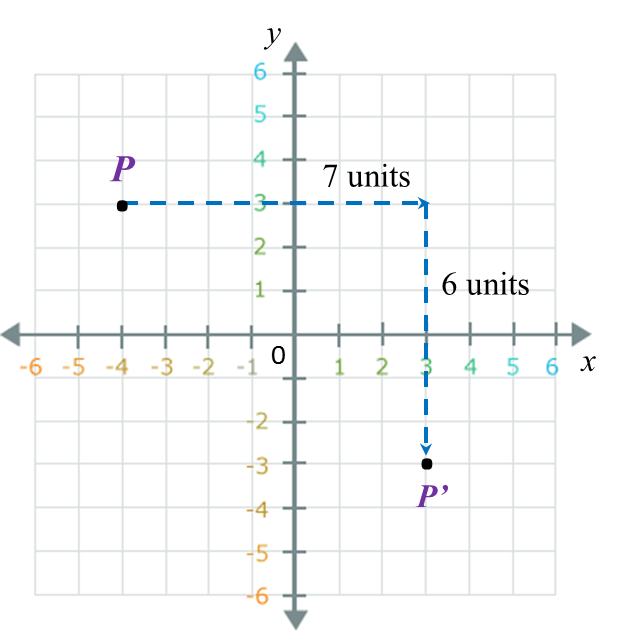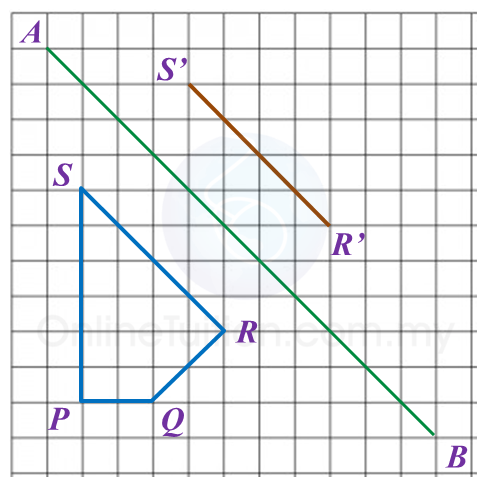# 11.2.1 Isometric Transformations, PT3 Focus Practice

      
      
         
11.2.1 Isometric Transformations, PT3 Focus Practice
         

 Question 1:
         
Diagram below in the answer space shows object drawn on a grid of equal squares with sides of 1 unit.
         
On the diagram, draw the image of object under the translation           $\left(\begin{array}{l}-6\\ \text{}3\end{array}\right).$          
         

     Answer
:Solution
:Question 2:
         
Describe the translation which maps point P onto point P’.Solution
:The translation is           $\left(\begin{array}{l}\text{}7\\ -6\end{array}\right).$          
 
    
 
     
       
         
Question 3:
         
Diagram below in the answer space shows quadrilateral PQRS. R’S’ is the image of RS under a reflection in the straight line AB.
         
On diagram in the answer space, complete the image of quadrilateral PQRS.
         

     Answer
:Solution: